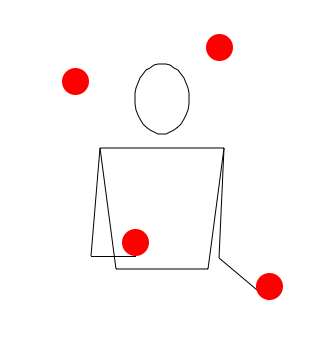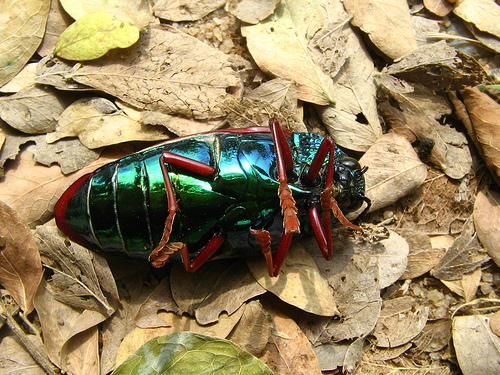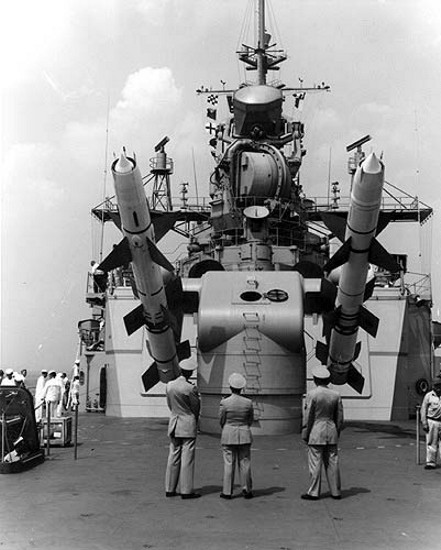Classical Mechanics

# 1D Kinematics: Level 3-4 ChallengesA juggler keeps $n$ balls going with one hand, so that at any instant, $n-1$ balls are in air and one ball is in his hand. If each ball arises to a height of $x$ meters, which of the following represents the time that each ball stays in his hand?

Recently there have been technological advances that allow for 3-d imaging up to kilometers away of an object. This can be done by using laser light that bounces off an object. By measuring the time it takes the laser light to get to the object and back, one can construct a detailed 3-d image of the object. The obstruction has been to get enough of the light back to actually make an image, but new detectors are sensitive enough to overcome this limitation.

If I want to measure a 3-d object down to millimeter accuracy, to what accuracy in seconds must my detector be able to measure the time of arrival of the light that bounced off the object?

Remember... the light has to bounce there and back.

Details and assumptions

• The speed of light is $3 \times 10^8~\mbox{m/s}$.There is a regular bus service between towns A and B, with a bus leaving towns A and B every T minutes. A cyclist moving with a speed of 20 [km/h] in the direction A to B notices that a bus goes past him every 18 minutes in the direction of him and every 6 minutes in the direction opposite direction. What is the period T (in minutes) of bus service?A scout from an ant colony (colony A) finds a dead beetle of mass $M$ a distance $d_A$ from his colony. At the same time, a scout from another colony (colony B) who is distance $d_B$ from his colony, also finds the beetle. If ants from colony B can walk at speed $v_B$, and workers from $A$ can each carry the mass $\delta m$ of ant flesh at any given time, what is the slowest speed (in m/s) at which workers from colony A walk so that workers from colony B never catch up and steal the beetle pieces?

Details

• If an ant from colony B catches an ant from colony A, it will kill the ant from colony A.
• If an ant from colony A makes it to colony A with beetle flesh, he is safe from workers from colony B.
• The scouts both start out at the beetle.
• $d_A=3$ m
• $d_B=5$ m
• $v_B=5$ m/s
• $M=250$ g
• $\delta m=0.5$ gA fighter plane is flying horizontally at a height of 250 m from ground with a constant velocity of 500 m/s. It passes exactly over a low-powered missile launcher which can fire a missile at any time in any direction with a speed of 100 m/s. Find the duration of time for which the plane is in danger of being hit by the missile in seconds.

Assumptions and Details

• Gravitational field strength at Earth's surface is 10 $m/s^2$

• The missile is not self-propelled

×